Скачать презентацию Arbitrage Pricing Theory Arbitrage Pricing Theory APT

616e01c15f571161bb803fc24865ee4c.ppt

• Количество слайдов: 6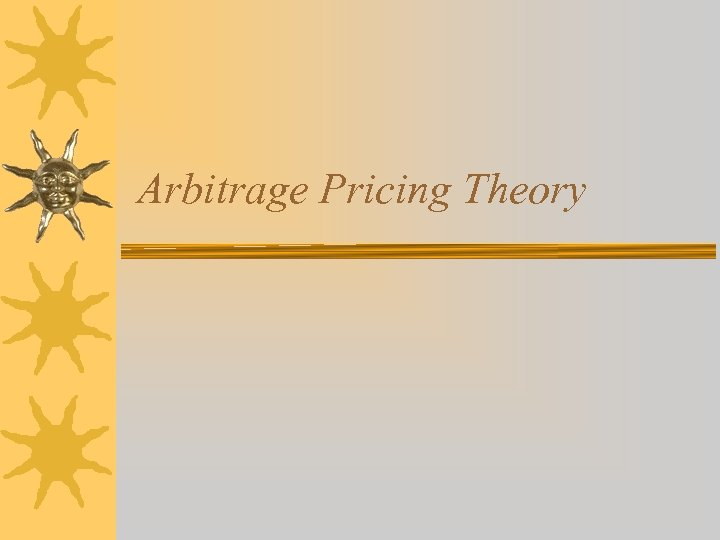Arbitrage Pricing Theory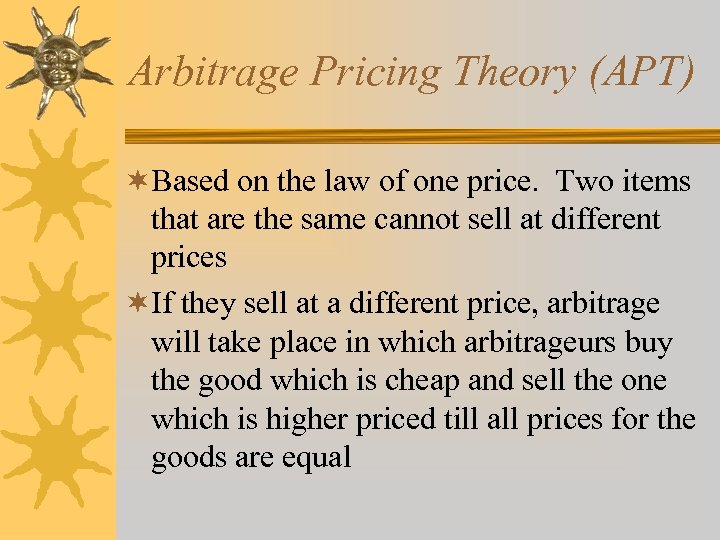Arbitrage Pricing Theory (APT) ¬Based on the law of one price. Two items that are the same cannot sell at different prices ¬If they sell at a different price, arbitrage will take place in which arbitrageurs buy the good which is cheap and sell the one which is higher priced till all prices for the goods are equal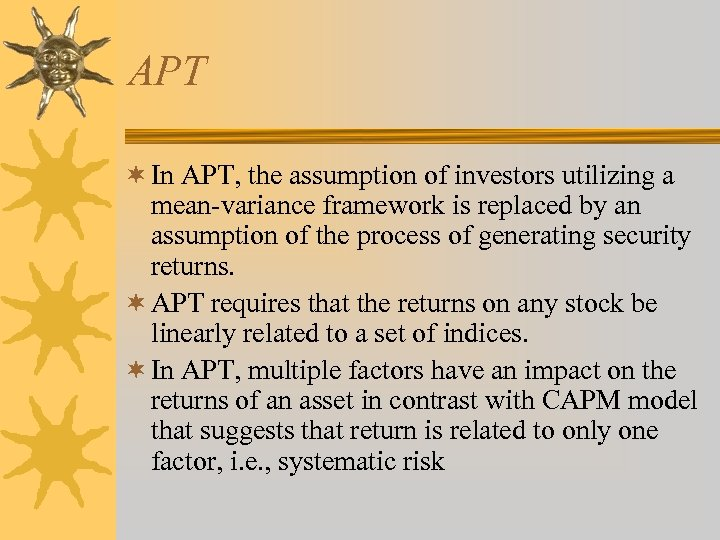APT ¬ In APT, the assumption of investors utilizing a mean-variance framework is replaced by an assumption of the process of generating security returns. ¬ APT requires that the returns on any stock be linearly related to a set of indices. ¬ In APT, multiple factors have an impact on the returns of an asset in contrast with CAPM model that suggests that return is related to only one factor, i. e. , systematic risk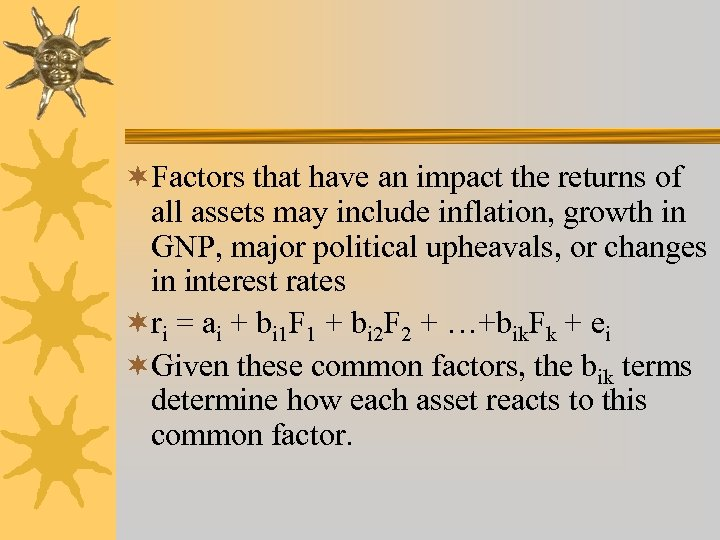¬Factors that have an impact the returns of all assets may include inflation, growth in GNP, major political upheavals, or changes in interest rates ¬ri = ai + bi 1 F 1 + bi 2 F 2 + …+bik. Fk + ei ¬Given these common factors, the bik terms determine how each asset reacts to this common factor.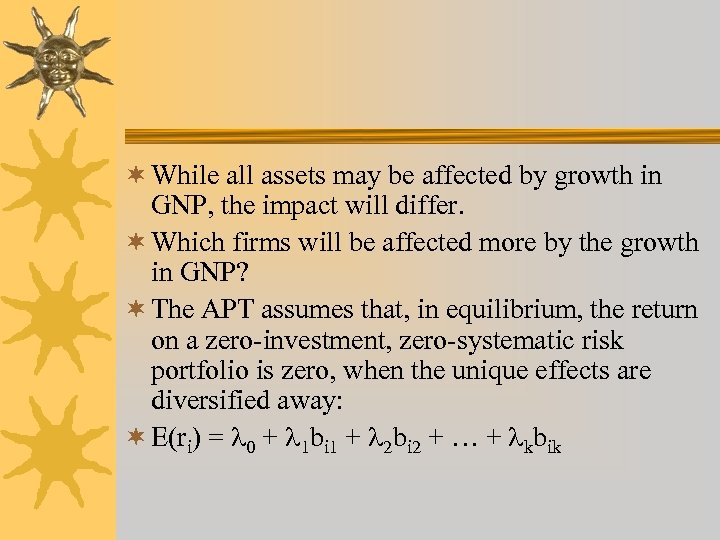¬ While all assets may be affected by growth in GNP, the impact will differ. ¬ Which firms will be affected more by the growth in GNP? ¬ The APT assumes that, in equilibrium, the return on a zero-investment, zero-systematic risk portfolio is zero, when the unique effects are diversified away: ¬ E(ri) = 0 + 1 bi 1 + 2 bi 2 + … + kbik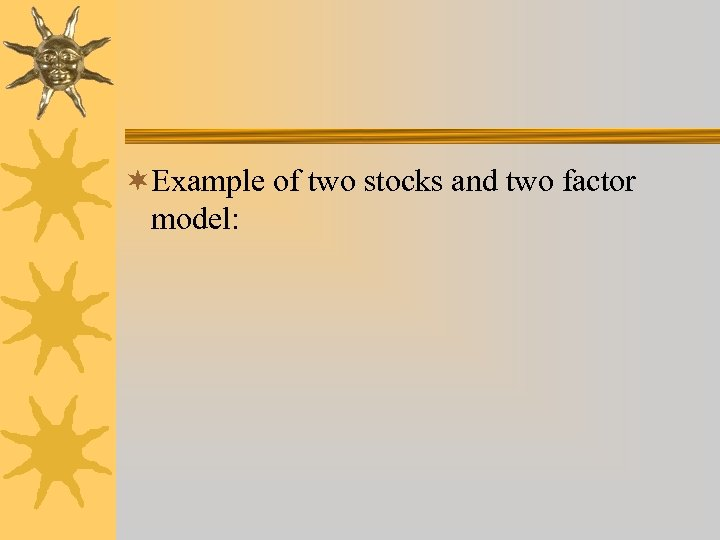¬Example of two stocks and two factor model: